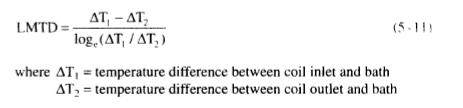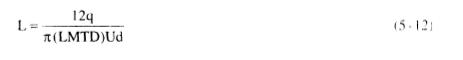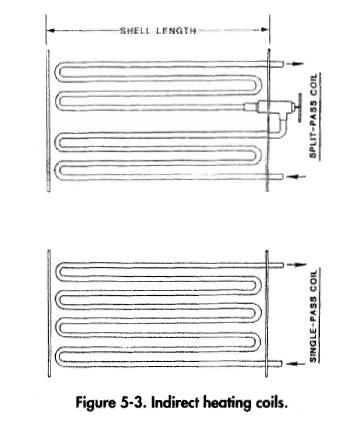﻿ LTX Coil Sizing – Coil Lengths | Oil & Gas Process Engineering

## LTX Coil Sizing – Coil Lengths

With the known temperatures on each end of the coil, the heat duty for each coil can be calculated from the heat transfer theory. Since the bath is at a constant temperature, LMTD can be calculated as:The overall film coefficient, U, for the coil can be calculated or read from the charts and tables in Heat Transfer Theory. Since U, LMTD, q, and the diameter of the pipe are known, the length of the coil can be solved from  the following equation:where d = coil outside diameter, in.

Equation 5-12 describes an overall length required for the coil. Since the shell length of the heater will probably be much less, several passes of the coil through the length of the shell may be required, as shown in Figure 5-3, to develop this length. For a given shell diameter there is a limit to the number of passes of coil. Therefore, the correct selection of coil length also requires determining the length of the shell and number of passes. As the shell length decreases, the number of passes increases, and a larger shell diameter is required.For a given shell length the number of passes for each coil can be determined. Since the number of passes both upstream and downstream of the choke must be an even integer, actual Tj and T2 may differ slightly from that assumed in the calculation. The actual values of Tj and T2 can be calculated from actual coil lengths LI and L2.

Once the total number of passes is known, the coil can be laid out geometrically assuming that the center-line minimum radius of bends is 11A times nominal pipe size. The required shell diameter is then determined.

Other selections of shell length, number of passes and required diameters can then be made to obtain an optimum solution.